# Appell polynomials

A class of polynomials over the field of complex numbers which contains many classical polynomial systems. The Appell polynomials were introduced by P.E. Appell . The series of Appell polynomialsis defined by the formal equality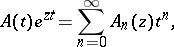(1)

where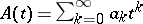is a formal power series with complex coefficients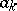,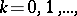and. The Appell polynomialshave an explicit expression in terms of the numbersas follows:The conditionis tantamount to saying that the degree of the polynomial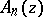is.

There is another, equivalent, definition of Appell polynomials. Let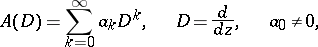be a differential operator, generally of infinite order, defined over the algebra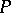of complex polynomials in the variable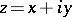. Theni.e.is the image of the functionunder the mapping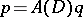,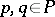.

The classof Appell polynomials is defined as the set of all possible systems of polynomialswith generating functions of the form (1). To say that a system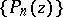of polynomials (of degree) belongs to the class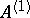amounts to saying that the relationships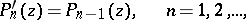are valid.

The Appell polynomials of classare sometimes defined bywhich, apart from normalization, are equivalent to those given above.

Appell polynomials of classare used to solve equations of the form:(2)

The formal equality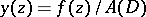for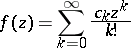makes it possible to write the solution of (2) in the formwhere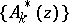are Appell polynomials with the generating function. In this connection the expansion of analytic functions into Appell polynomials is of special interest. Appell polynomials also find use in various problems connected with functional equations, including differential equations other than (2), in interpolation problems, in approximation theory, in summation methods, etc. (cf. ). For a more general account of the theory of Appell polynomials of class, and a number of applications, see .

The classcontains, as special cases, a large number of classical sequences of polynomials. Examples, up to a normalization, are the Bernoulli polynomials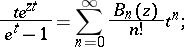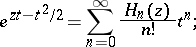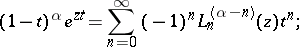etc. For many other examples, see  and , Vol. 3.

There exist various generalizations of Appell polynomials, which are also known as systems of Appell polynomials. These include the Appell polynomials with generating functions of the form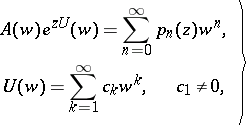(3)

and the Appell polynomials with the more general generating functions: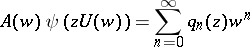(4)

(see, for example, , , Vol. 3). Ifis the inverse function to the function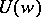, then the fact that the system of polynomials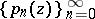belongs to the class of sequences of Appell polynomials with a generating function of type (3) is equivalent to the validity of the relations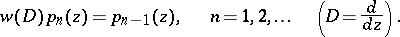There are only five weighted orthogonal systems of sequences of Appell polynomials on the real axis with generating functions of the type (3); these include only one orthogonal system with generating functions of the type (1), which consists of Hermite polynomials with the weight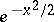on the real axis (cf. ).

For the expansion in series by Appell polynomials with generating functions of the types (3) and (4), and interconnections of these polynomials by various functional equations see , , .

The class, whereis an integer, of Appell polynomials is defined as follows: It is the set of all systems of polynomials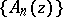for each of which the (formal) representationis valid. Here,, and,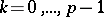, are formal power series, the free terms of which are such that the degree of the polynomialis. To say that a sequence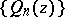of polynomials of degreebelongs to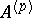amounts to saying that the relations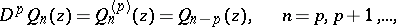are valid. For problems on the expansion of analytic functions in series by Appell polynomials of class, see . They are closely connected with the problem of finding analytic solutions of functional equations of the typeAppell polynomials in two variables were introduced by P. Appell . They are defined by the equations: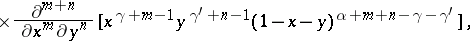in which it is assumed that,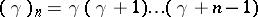for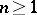; these Appell polynomials are analogues of the Jacobi polynomials. The Appell polynomials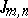are orthogonal with the weight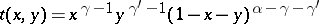(5)

to any polynomials in two variables of degree lower than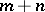, over the domain, whereis the triangle:,,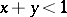; however, they do not form a system of orthogonal functions with the weight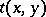in(see, for example, , Vol. 2).